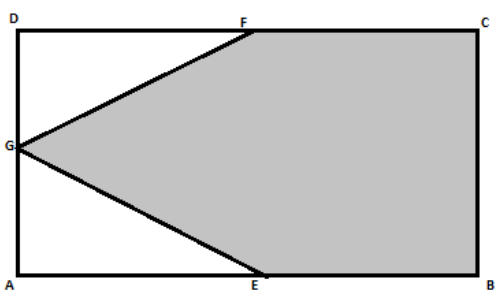QUESTION

# Calculate the area of the shaded region of the following figure. ABCD is a rectangle having length 30 cm, breadth 20 cm. E, F and G are midpoints of AB, CD and AD respectively.Hint: Here, we can first calculate the total area of the rectangle ABCD by using the formula for the area of a rectangle which is $A=length\times breadth$ and then we can subtract the area of the triangles FDG and EAG to get the area of the shaded region.

We know that the formula for the area of a rectangle is given as:
$A=length\times breadth..........\left( 1 \right)$
Using this formula we can find the area of the rectangle as:
\begin{align} & A=AB\times BC \\ & \Rightarrow A=30cm\times 20cm \\ & \Rightarrow A=600c{{m}^{2}} \\ \end{align}
Since, it is given that E, F and G are the midpoints of AB, CD and AD respectively.
So, the length of DF will be half of the length of the CD. So, we have:
\begin{align} & DF=\frac{1}{2}\times CD \\ & \Rightarrow DF=\frac{1}{2}\times 30cm \\ & \Rightarrow DF=15cm \\ \end{align}
Since, we have AB = CD, on dividing both sides by 2 we get:
$\dfrac{AB}{2}=\dfrac{CD}{2}$
Therefore, AE = DF =15 cm
Similarly, we also have AD = 20 cm and G is the midpoint of AD. So, we have:
$DG=AG=\dfrac{20cm}{2}=10cm$
We know that all the angles in a rectangle are equal to 90degrees. It means that triangle FDG and triangle EAG are right angled triangles.
So, here we can use the formula for the area of a right angled triangle which is given as:
$A=\dfrac{1}{2}\times base\times height$
So, using this formula area of triangles FDG and EAG can be found as:
\begin{align} & \text{Area of }\Delta \text{FDG=}\frac{1}{2}\times DG\times DF \\ & \Rightarrow \text{Area of }\Delta \text{FDG}=\frac{1}{2}\times 10\times 15=75c{{m}^{2}} \\ \end{align}
So, the area of triangle FDG is = $75c{{m}^{2}}$
Also,
\begin{align} & \text{Area of }\Delta EAG\text{=}\frac{1}{2}\times AG\times AE \\ & \Rightarrow \text{Area of }\Delta EA\text{G}=\frac{1}{2}\times 10\times 15=75c{{m}^{2}} \\ \end{align}
So, the area of the triangle EAG is also equal to $75c{{m}^{2}}$.
Therefore, the area of the shaded region is:
= area of rectangle ABCD – (area of triangle FDG + area of triangle EAG)
\begin{align} & =600c{{m}^{2}}-\left( 75c{{m}^{2}}+75c{{m}^{2}} \right) \\ & =600c{{m}^{2}}-150c{{m}^{2}} \\ & =450c{{m}^{2}} \\ \end{align}
So, the area of the shaded region is equal to $450c{{m}^{2}}$.
Hence, option (b) is the correct answer.

Note: Students should note that the triangles FDG and EAG are both right angled triangles. It is because all the angles of a rectangle are 90 degrees.